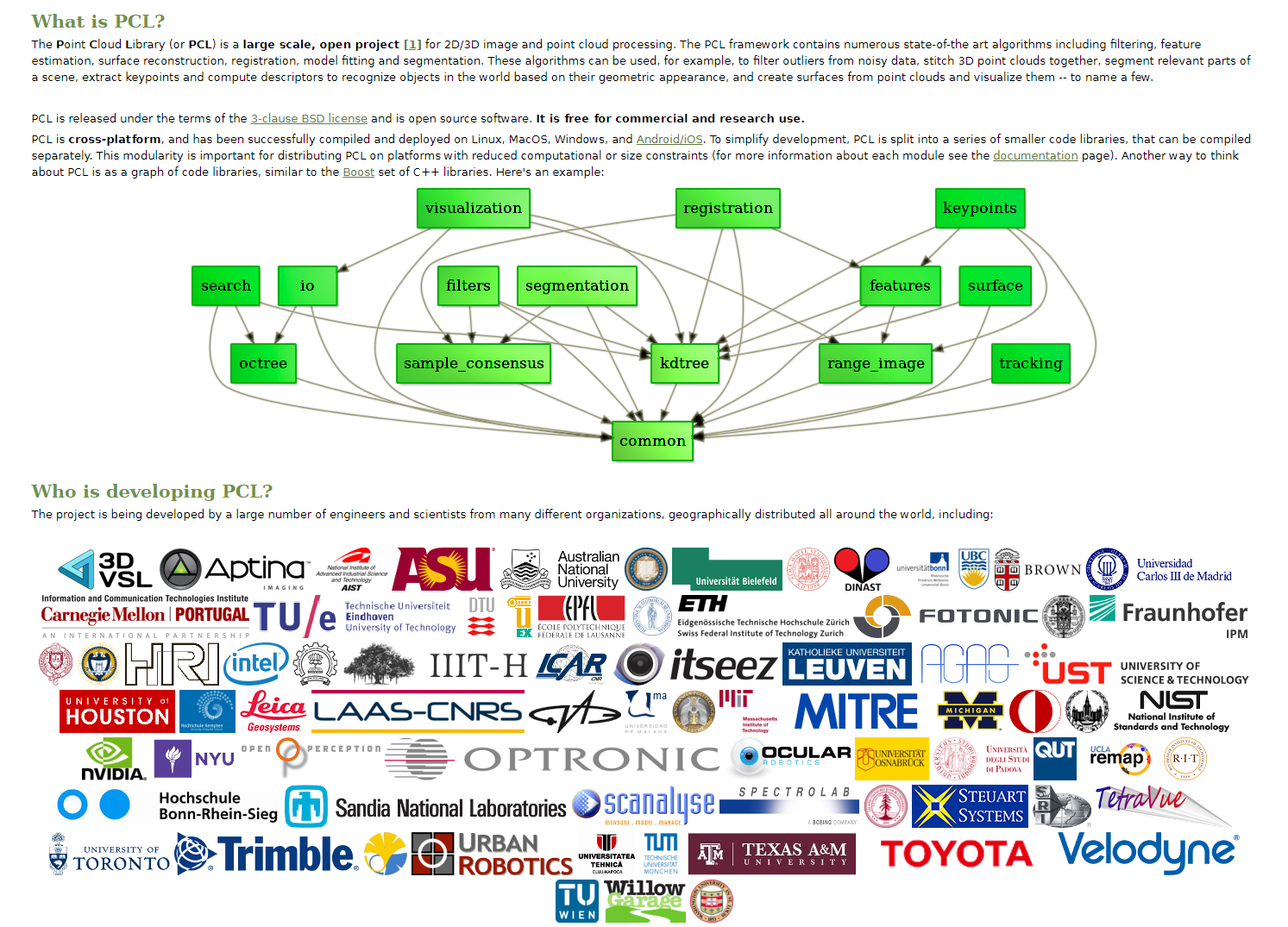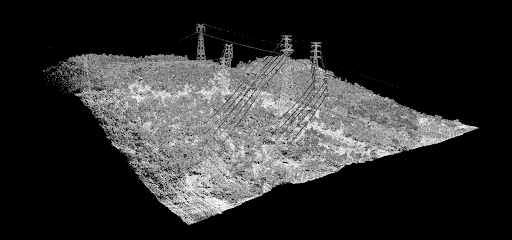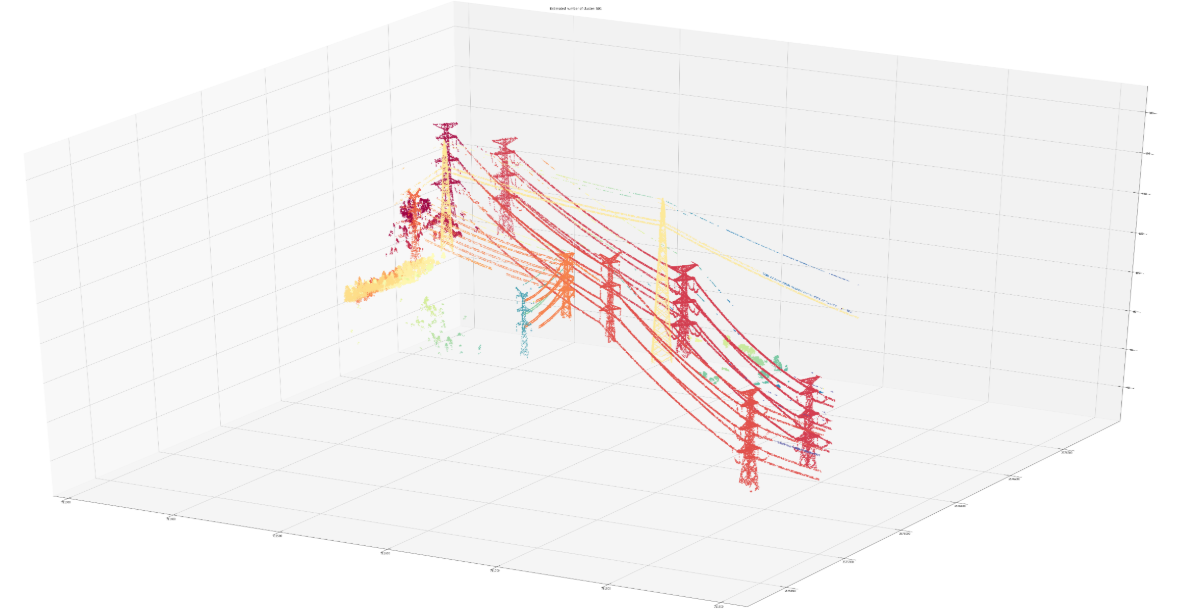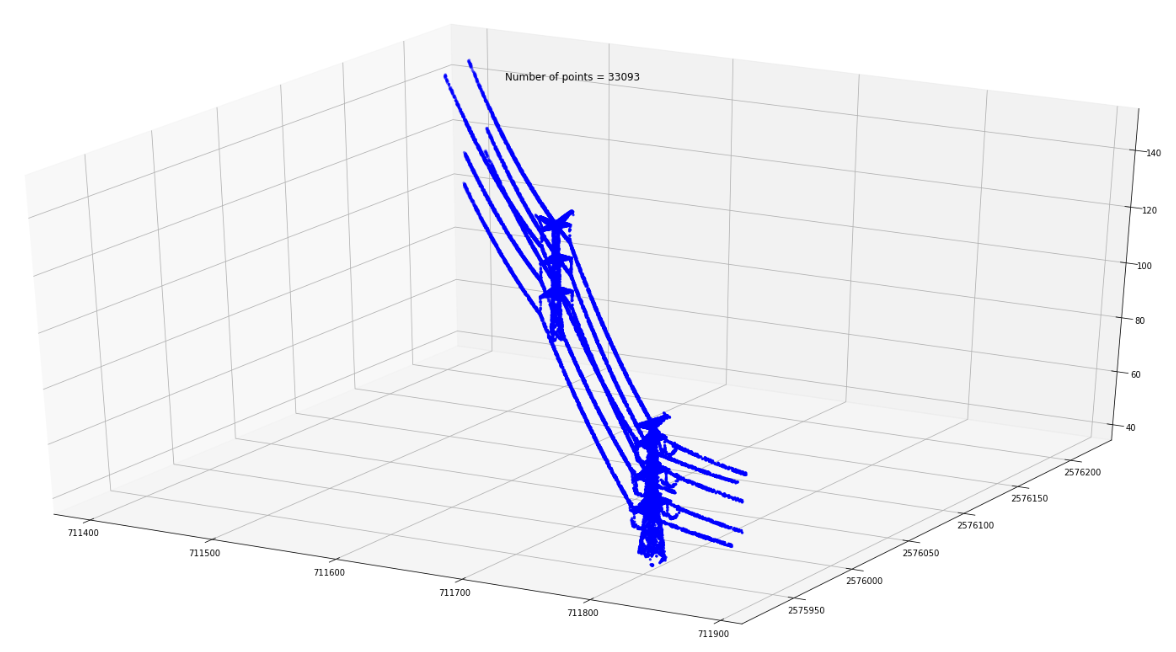## Introduction to Point Cloud Data

In recent years, there was great progress in the development of LIDAR detectors that resulted in huge amounts of raw data. LIDARs are now more accurate and provide a much higher resolution than even 10 years ago. Aerial-based LIDARs have become an efficient method for receiving information about the environment. Nevertheless, the data you get is in fact only a collection of sparse points that may provide some nice visualization but require extensive processing when it comes to more practical purposes. Unfortunately, as of now, the progress of computer vision mostly concerns structured two-dimensional data (photo, video). The current methods do not generalize to multidimensional sparse data like Point Cloud that we receive after a basic LIDAR data pre-processing. Extensive research is being done in the field. Weshould particularly mention PCL – a graf of libraries developed by a great international community to provide instruments for both 2D and 3D data for a wide variety of applications. Unfortunately, at the moment it is not an easy task to apply the library to some solution of interest. It often means that you have to dive into the library too deep. It makes sense for production-grade products that need high scalability. But it may be too costly for a PoC development. Therefore, we decided to try what can be done with point cloud data using a simple approach and pretty standard Python libraries (PCL can be used from Python but only so far, since only small subsets can be integrated seamlessly).

## Data for the Experiment

As an example let’s use the data generated by an aerial-based LIDAR for the detection of power lines. Power lines are often clearly visible in point cloud visualization. However, mapping the points that belong to a power line requires a lot of manual efforts. On the other hand, simple geometrical consideration may provide us with means to greatly simplify or even automate such processing.

The power line on the picture is actually a collection of points which form certain geometrical patterns. To simplify further categorization, I decided to check how we can form clusters out of these points.## Software Used for the Experiment in Point Cloud Data

I will use NumPy, Sklearn, Laspy and SciPy libraries for the purpose of cluster formation and matplotlib for visualization.

```import laspy
import scipy
import numpy as np
import matplotlib.pyplot as plt
from sklearn.cluster import DBSCAN
from sklearn import metrics
from sklearn import preprocessing
from mpl_toolkits.mplot3d import Axes3D
from matplotlib import path```

Laspy is great for handling point cloud data in Python. We read point cloud data from a las file and check the shape of the actual dataset.

```# Open a file in read mode:
inFile = laspy.file.File(“./LAS/simple.las”)
# Grab a numpy dataset of our clustering dimensions:
dataset = np.vstack([inFile.x, inFile.y, inFile.z]).transpose()
dataset.shape```

(5942479, 3) — our point cloud consists of 5942479 points. It is not enough if you want to get to small details. But the number is too big if you try to convert this DataFrame into a three-dimensional NumPy array, as in this case, we will get a huge 5942479³ = 2.09*10²⁰ array. It will use an enormous amount of RAM for the storage of really sparse data. The obvious thing to do is to use a NumPy sparse array. But as a matter of fact, a sparse array works great for 2D but fails with 3D data. Matrix manipulation functions are not fully compatible with sparse 3D matrices. We have to stick to working with a DataFrame instead of a NumPy array due to memory requirements.

## Eliminating Out-of-Scope Points

We need to find a method to eliminate those points that are not power lines. Power lines are placed high above the ground for safety reasons and to ensure their optimal performance. But for the rugged terrain, we have to take into account that some ground points can be higher than power lines in different parts of the image due to the ground incline. To avoid this let’s divide our point cloud to small vertical parts.

```%%time
def frange(start, stop, step):
i = start
while i < stop:
yield i
i += step
#ground points grid filter
n = 100 #grid step
dataset_Z_filtered = dataset[]
zfiltered = (dataset[:, 2].max() — dataset[:, 2].min())/10 #setting height filtered from ground
print(‘zfiltered =’, zfiltered)
xstep = (dataset[:, 0].max() — dataset[:, 0].min())/n
ystep = (dataset[:, 1].max() — dataset[:, 1].min())/n
for x in frange (dataset[:, 0].min(), dataset[:, 0].max(), xstep):
for y in frange (dataset[:, 1].min(), dataset[:, 1].max(), ystep):
datasetfiltered = dataset[(dataset[:,0] > x)
&(dataset[:, 0] < x+xstep)
&(dataset[:, 1] > y)
&(dataset[:, 1] < y+ystep)]
if datasetfiltered.shape > 0:
datasetfiltered = datasetfiltered[datasetfiltered[:, 2]
>(datasetfiltered[:, 2].min()+ zfiltered)]
if datasetfiltered.shape > 0:
dataset_Z_filtered = np.concatenate((dataset_Z_filtered,
datasetfiltered))
print(‘dataset_Z_filtered shape’, dataset_Z_filtered.shape)```

With the help of this simple approach, we can greatly reduce the number of points in the cloud in no time even using moderate computing power. In our case, it was the reduction of the number of points by an order of magnitude in 3 minutes — not bad for a few lines of code, given that we made no real efforts for any optimization.

```dataset_Z_filtered shape (169862, 3)
CPU times: user 3min 16s, sys: 7.14 ms, total: 3min 16s
Wall time: 3min 16s```

Now we’ll be using a much smaller filtered dataset.

## Exploring Our Data for Point Cloud

Let’s explore our data:

```print(“Examining Point Format: “)
pointformat = inFile.point_format
for spec in inFile.point_format:
print(spec.name)
Examining Point Format:
X
Y
Z
intensity
flag_byte
raw_classification
scan_angle_rank
user_data
pt_src_id
gps_time```

During my experiments, I try to use the 4D representation of data (X, Y, Z, and intensity) but the results do not improve over 3D (X, Y, Z) so let’s stick to the latter subset of data.

```print(‘Z range =’, dataset[:, 2].max() — dataset[:, 2].min())
print(‘Z max =’, dataset[:, 2].max(), ‘Z min =’, dataset[:, 2].min())
print(‘Y range =’, dataset[:, 1].max() — dataset[:, 1].min())
print(‘Y max =’, dataset[:, 1].max(), ‘Y min =’, dataset[:, 1].min())
print(‘X range =’, dataset[:, 0].max() — dataset[:, 0].min())
print(‘X max =’, dataset[:, 0].max(), ‘X min =’, dataset[:, 0].min())
Z range = 149.81
Z max = 181.78999908447264 Z min = 31.979999084472652
Y range = 622.9700000002049
Y max = 2576396.509974365 Y min = 2575773.539974365
X range = 556.4400000000605
X max = 711882.7199987792 X min = 711326.2799987792```

## Data Normalization

As you can see, the values are in different ranges. For better results, we should normalize the dataset.

`dataset = preprocessing.normalize(dataset)`

## Clustering the Points That Are Geometrically Close

Now we are ready to process our data. Our power lines are actually spatial clusters of points so it is natural to try a clustering algorithm. After a short investigation, I found that DBSCAN provided by the Sklearn library works best out-of-the-box.

`clustering = DBSCAN(eps=2, min_samples=5, leaf_size=30).fit(dataset)`

Now let’s visualize our results.

```core_samples_mask = np.zeros_like(clustering.labels_, dtype=bool)
labels = clustering.labels_
# Number of clusters in labels, ignoring noise if present.
n_clusters_ = len(set(labels)) — (1 if -1 in labels else 0)
n_noise_ = list(labels).count(-1)
print(‘Estimated number of clusters: %d’ % n_clusters_)
print(‘Estimated number of noise points: %d’ % n_noise_)
Estimated number of clusters: 501
Estimated number of noise points: 1065```

## Visualization

Most of our points were grouped into clusters. Let’s see what it looks like in practice:

```# Black removed and is used for noise instead.
fig = plt.figure(figsize=[100, 50])
unique_labels = set(labels)
colors = [plt.cm.Spectral(each)
for each in np.linspace(0, 1, len(unique_labels))]
for k, col in zip(unique_labels, colors):
if k == -1:
# Black used for noise.
col = [0, 0, 0, 1]
ax.scatter(xyz[:, 0], xyz[:, 1], xyz[:, 2], c=col, marker=”.”)
plt.title(‘Estimated number of cluster: %d’ % n_clusters_)
plt.show()```

It is clear now that simple geometrical consideration and a pretty standard clustering method help us to simplify the point categorization using moderate computing resources.

Each cluster of points can be categorized separately if needed.### Results

Our experiment showed that a combination of geometrical consideration and standard Python libraries can result in a significant reduction of efforts needed to categorize raw point cloud data for further usage.

Author: Alex Simkiv, Data Scientist|Co-founder at MindCraft Information Technology & Data Science Read Also: Object Detection and Counting